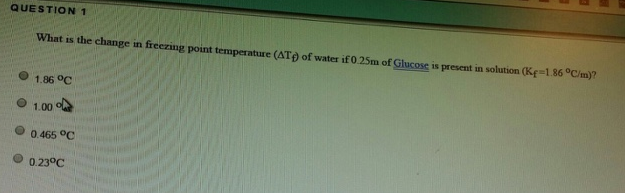# Problem: What is the change in freezing point temperature (ΔTf) of water if 0.25 m of Glucose is present in solution (Kf = 1.86°C/m)?a. 1.86°Cb. 1.00°Cc. 0.465°Cd. 0.23°C

###### FREE Expert Solution

ΔT of Freezing point depression can be solved using

96% (44 ratings)###### Problem Details

What is the change in freezing point temperature (ΔTf) of water if 0.25 m of Glucose is present in solution (Kf = 1.86°C/m)?

a. 1.86°C

b. 1.00°C

c. 0.465°C

d. 0.23°C